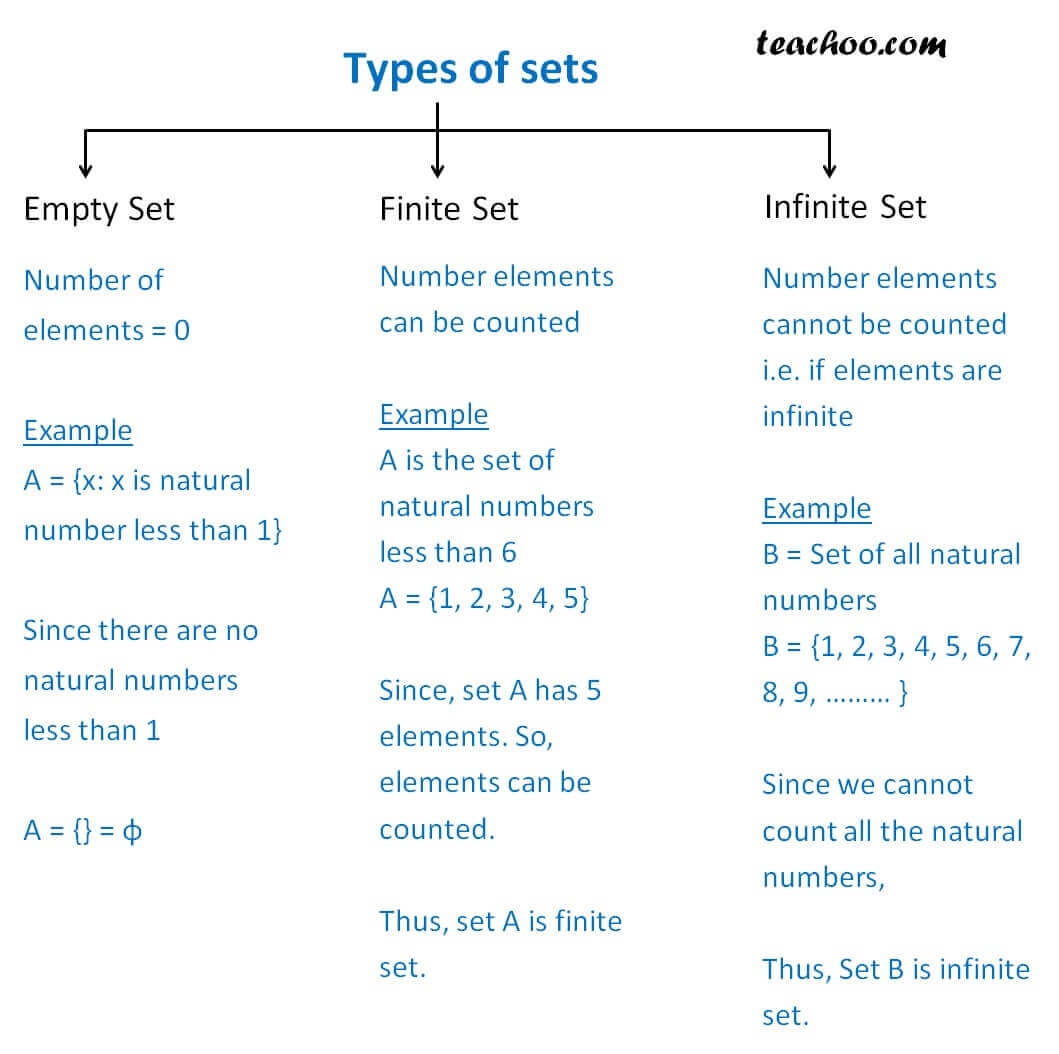Finite/Infinite

Chapter 1 Class 11 Sets
Concept wise

There different types of sets

Let us compare themGet live Maths 1-on-1 Classs - Class 6 to 12

### Transcript

Types of sets Empty Set Number of elements = 0 Example A = {x: x is natural number less than 1} Since there are no natural numbers less than 1 A = {} = φ Finite Set Number elements can be counted Example A is the set of natural numbers less than 6 A = {1, 2, 3, 4, 5} Since, set A has 5 elements. So, elements can be counted. Thus, set A is finite set. Infinite Set Number elements cannot be counted i.e. if elements are infinite Example B = Set of all natural numbers B = {1, 2, 3, 4, 5, 6, 7, 8, 9, ……… } Since we cannot count all the natural numbers, Thus, Set B is infinite set.